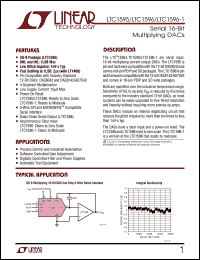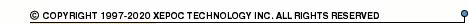More than4 307 195 components listedLTC1595ACN8 series datasheets. Manufacturer: Linear Technology.

 LTC1595ACN8 Serial 16-bit multiplying DACs in 8-pin PDIP package. Operational temperature range from 0°C to 70°C. Datasheet*) LTC1595ACS8 Serial 16-bit multiplying DACs in 8-pin SO package. Operational temperature range from 0°C to 70°C. Datasheet*) LTC1595AIN8 Serial 16-bit multiplying DACs in 8-pin PDIP package. Operational temperature range from -40°C to 85°C. Datasheet*) LTC1595AIS8 Serial 16-bit multiplying DACs in 8-pin SO package. Operational temperature range from -40°C to 85°C. Datasheet*) LTC1595BCN8 Serial 16-bit multiplying DACs in 8-pin PDIP package. Operational temperature range from 0°C to 70°C. Datasheet*) LTC1595BCS8 Serial 16-bit multiplying DACs in 8-pin SO package. Operational temperature range from 0°C to 70°C. Datasheet*) LTC1595BIN8 Serial 16-bit multiplying DACs in 8-pin PDIP package. Operational temperature range from -40°C to 85°C. Datasheet*) LTC1595BIS8 Serial 16-bit multiplying DACs in 8-pin SO package. Operational temperature range from -40°C to 85°C. Datasheet*) LTC1595CCN8 Serial 16-bit multiplying DACs in 8-pin PDIP package. Operational temperature range from 0°C to 70°C. Datasheet*) LTC1595CCS8 Serial 16-bit multiplying DACs in 8-pin SO package. Operational temperature range from 0°C to 70°C. Datasheet*) LTC1595CIN8 Serial 16-bit multiplying DACs in 8-pin PDIP package. Operational temperature range from -40°C to 85°C. Datasheet*) LTC1595CIS8 Serial 16-bit multiplying DACs in 8-pin SO package. Operational temperature range from -40°C to 85°C. Datasheet*) LTC1596-1ACN Serial 16-bit multiplying DACs in 16-pin PDIP package. Operational temperature range from 0°C to 70°C. Datasheet*) LTC1596-1ACSW Serial 16-bit multiplying DACs in 16-pin SO package. Operational temperature range from 0°C to 70°C. Datasheet*) LTC1596-1AIN Serial 16-bit multiplying DACs in 16-pin PDIP package. Operational temperature range from -40°C to 85°C. Datasheet*) LTC1596-1AISW Serial 16-bit multiplying DACs in 16-pin SO package. Operational temperature range from -40°C to 85°C. Datasheet*) LTC1596-1BCN Serial 16-bit multiplying DACs in 16-pin PDIP package. Operational temperature range from 0°C to 70°C. Datasheet*) LTC1596-1BCSW Serial 16-bit multiplying DACs in 16-pin SO package. Operational temperature range from 0°C to 70°C. Datasheet*) LTC1596-1BIN Serial 16-bit multiplying DACs in 16-pin PDIP package. Operational temperature range from -40°C to 85°C. Datasheet*) LTC1596-1BISW Serial 16-bit multiplying DACs in 16-pin SO package. Operational temperature range from -40°C to 85°C. Datasheet*) LTC1596-1CCN Serial 16-bit multiplying DACs in 16-pin PDIP package. Operational temperature range from 0°C to 70°C. Datasheet*) LTC1596-1CCSW Serial 16-bit multiplying DACs in 16-pin SO package. Operational temperature range from 0°C to 70°C. Datasheet*) LTC1596-1CIN Serial 16-bit multiplying DACs in 16-pin PDIP package. Operational temperature range from -40°C to 85°C. Datasheet*) LTC1596-1CISW Serial 16-bit multiplying DACs in 16-pin SO package. Operational temperature range from -40°C to 85°C. Datasheet*) LTC1596ACN Serial 16-bit multiplying DACs in 16-pin PDIP package. Operational temperature range from 0°C to 70°C. Datasheet*) LTC1596ACSW Serial 16-bit multiplying DACs in 16-pin SO package. Operational temperature range from 0°C to 70°C. Datasheet*) LTC1596AIN Serial 16-bit multiplying DACs in 16-pin PDIP package. Operational temperature range from -40°C to 85°C. Datasheet*) LTC1596AISW Serial 16-bit multiplying DACs in 16-pin SO package. Operational temperature range from -40°C to 85°C. Datasheet*) LTC1596BCN Serial 16-bit multiplying DACs in 16-pin PDIP package. Operational temperature range from 0°C to 70°C. Datasheet*) LTC1596BCSW Serial 16-bit multiplying DACs in 16-pin SO package. Operational temperature range from 0°C to 70°C. Datasheet*) LTC1596BIN Serial 16-bit multiplying DACs in 16-pin PDIP package. Operational temperature range from -40°C to 85°C. Datasheet*) LTC1596BISW Serial 16-bit multiplying DACs in 16-pin SO package. Operational temperature range from -40°C to 85°C. Datasheet*) LTC1596CCN Serial 16-bit multiplying DACs in 16-pin PDIP package. Operational temperature range from 0°C to 70°C. Datasheet*) LTC1596CCSW Serial 16-bit multiplying DACs in 16-pin SO package. Operational temperature range from 0°C to 70°C. Datasheet*) LTC1596CIN Serial 16-bit multiplying DACs in 16-pin PDIP package. Operational temperature range from -40°C to 85°C. Datasheet*) LTC1596CISW Serial 16-bit multiplying DACs in 16-pin SO package. Operational temperature range from -40°C to 85°C. Datasheet*)